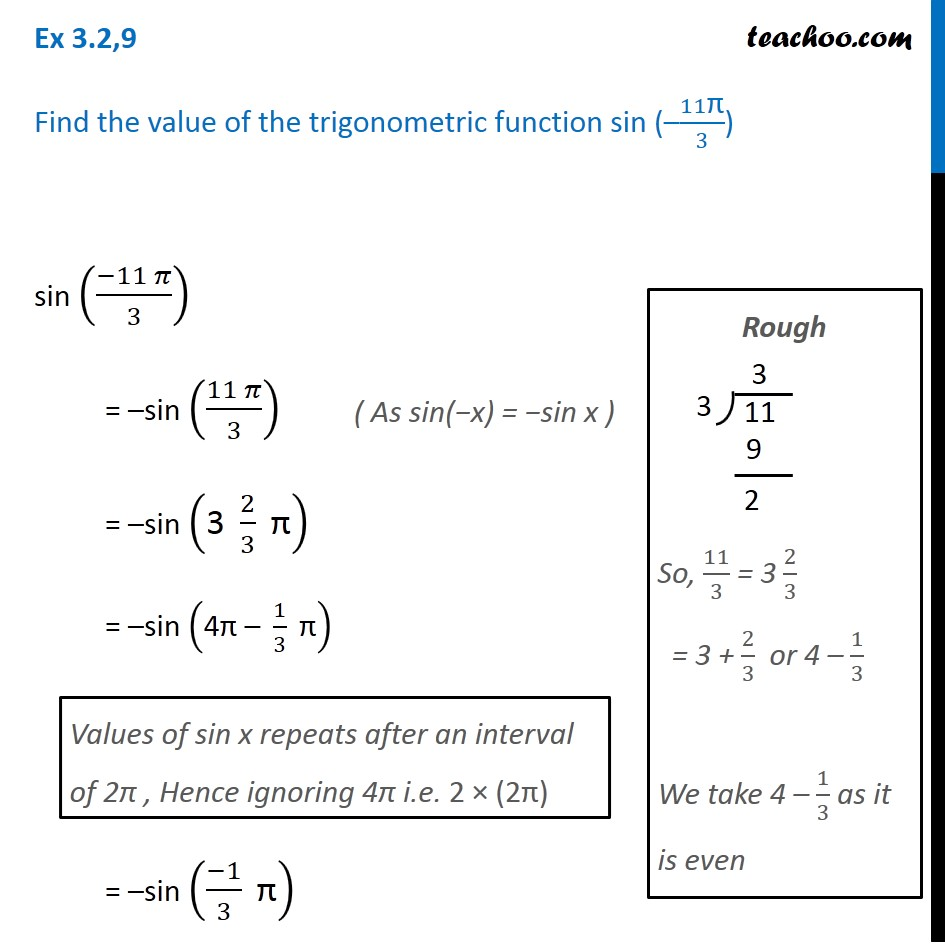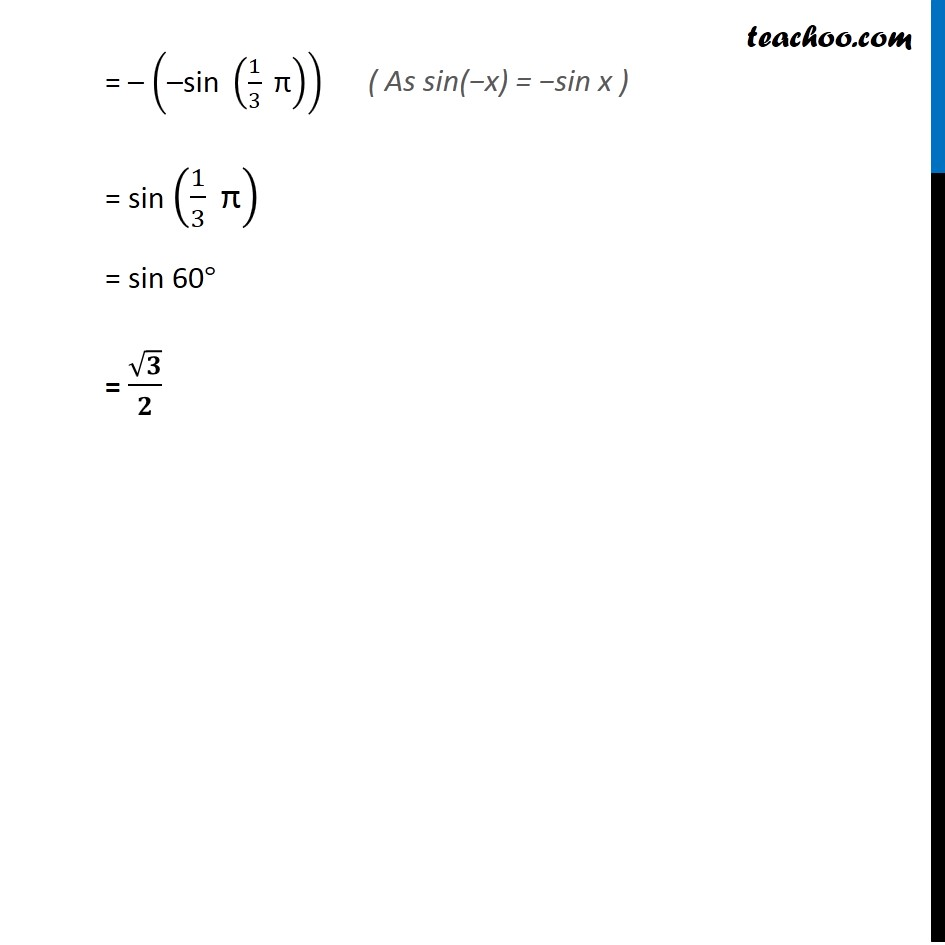Learn All Concepts of Chapter 2 Class 11 Relations and Function - FREE. Check - Trigonometry Class 11 - All Concepts1. Chapter 3 Class 11 Trigonometric Functions
2. Concept wise
3. Finding Value of trignometric functions, given angle

Transcript

Ex 3.2,9 Find the value of the trigonometric function sin (–11"π" /3) sin ((−11 𝜋)/3) = –sin ((11 𝜋)/3) = –sin ("3 " 2/3 " π" ) = –sin ("4π – " 1/3 " π" ) = –sin ((−1)/3 " π" ) Values of sin x repeats after an interval of 2π , Hence ignoring 4π i.e. 2 × (2π) Rough So, 11/3 = 3 2/3 = 3 + 2/3 or 4 – 1/3 We take 4 – 1/3 as it is even = – ("–sin " (1/3 " π" )) = sin (1/3 " π" ) = sin 60° = √𝟑/𝟐 ( As sin(−x) = −sin x )

Finding Value of trignometric functions, given angle

About the AuthorDavneet Singh
Davneet Singh is a graduate from Indian Institute of Technology, Kanpur. He has been teaching from the past 9 years. He provides courses for Maths and Science at Teachoo.